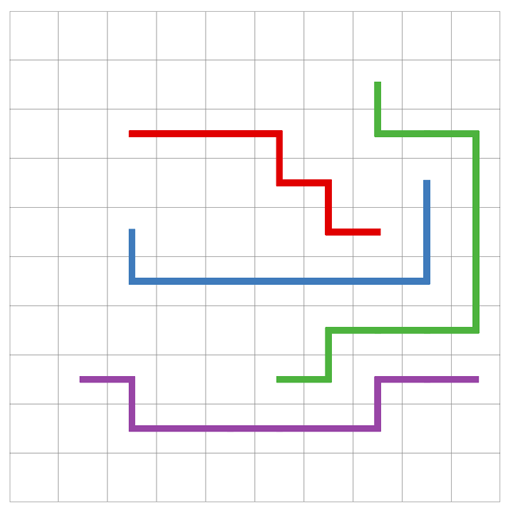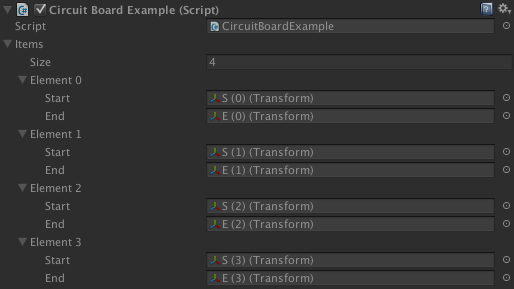A* Pathfinding Project  4.1.2 The A* Pathfinding Project for Unity 3D
CircuitBoardExample.cs

This example shows how to use the Pathfinding.ITraversalProvider interface to generate paths like on a circuit-board.I.e paths that do not share any node. The image below shows a test case when using the script to calculate 4 paths on a small grid. The visualization of the paths has been improved manually using an external photo-editing application.The code has intentionally been left simple, so very little error checking and special case handling is done.

Note that finding paths on a circuit-board in an optimal way is a very hard problem (NP-Complete). For further information about that, see https://en.wikipedia.org/wiki/Multi-commodity_flow_problem.

Attach this script to any GameObject and fill in the 'items' array with the start end endpoints of each path.Utilities for turn-based games
Pathfinding.ITraversalProvider
﻿using UnityEngine;
using System.Collections.Generic;
using Pathfinding;
public class CircuitBoardExample : MonoBehaviour {
[System.Serializable]
public class Item {
public Transform start;
public Transform end;
}
public Item[] items;
class Blocker : ITraversalProvider {
public HashSet<GraphNode> blockedNodes = new HashSet<GraphNode>();
public bool CanTraverse (Path path, GraphNode node) {
// Override the default logic of which nodes can be traversed
return DefaultITraversalProvider.CanTraverse(path, node) && !blockedNodes.Contains(node);
}
public uint GetTraversalCost (Path path, GraphNode node) {
// Use the default costs
return DefaultITraversalProvider.GetTraversalCost(path, node);
}
}
void Update () {
var traversalProvider = new Blocker();
// Calculate all paths in sequence.
// It is important that they are not calculated in parallel
// as we need to make sure that the paths do not visit the same nodes.
// The result may vary significantly depending on the order in which
// the paths are calculated.
for (int index = 0; index < items.Length; index++) {
var item = items[index];
// Create new path object
ABPath path = ABPath.Construct(item.start.position, item.end.position);
path.traversalProvider = traversalProvider;
// Start calculating the path and put the path at the front of the queue
AstarPath.StartPath(path, true);
// Calculate the path immediately
path.BlockUntilCalculated();
// Make sure the remaining paths do not use the same nodes as this one
foreach (var node in path.path) {